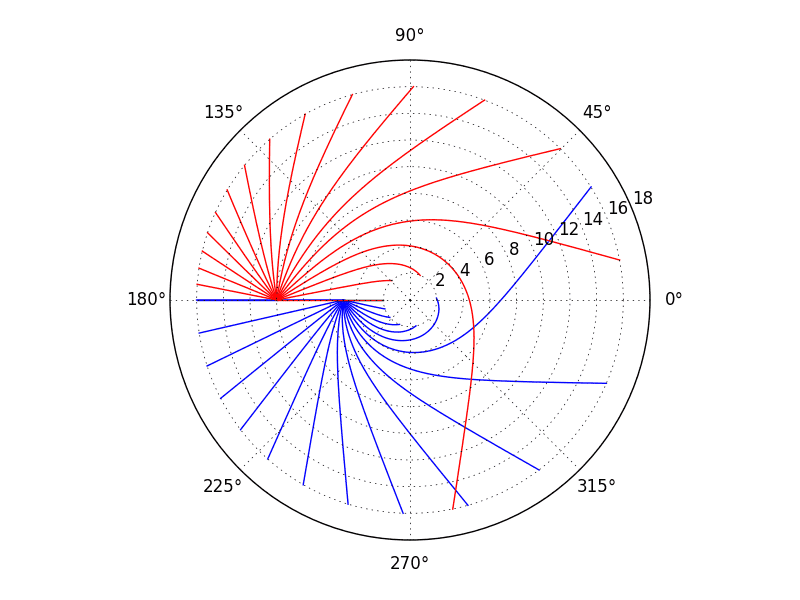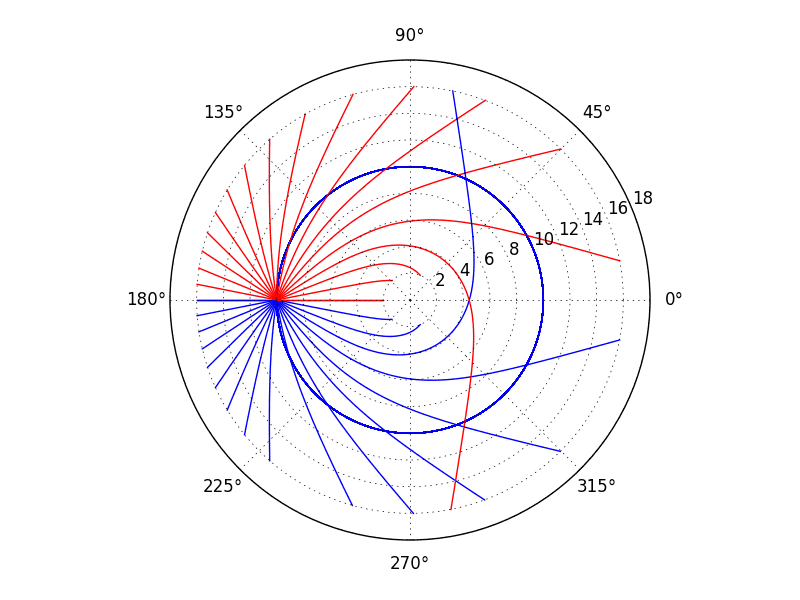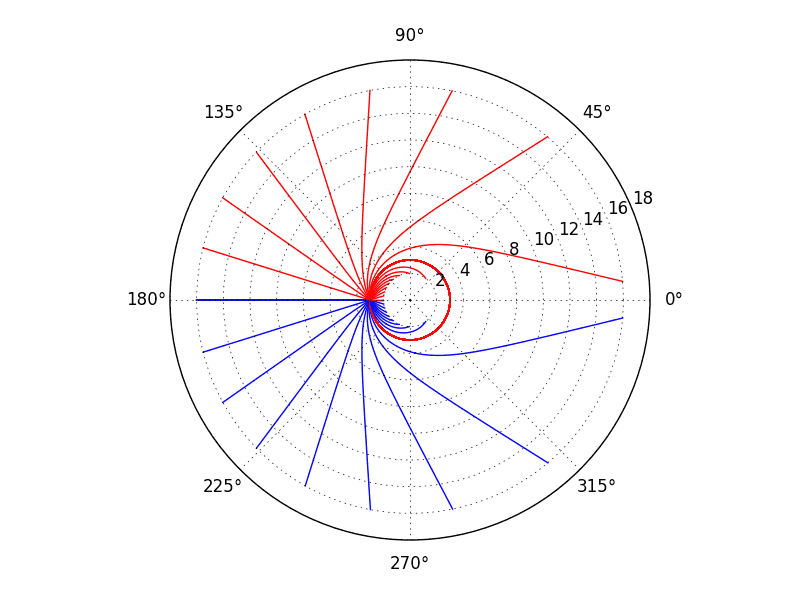# Null geodesics in Schwarzschild spacetime

• I
I was looking at null geodesics in Schwarzschild spacetime. Carroll's lecture notes cover them here: https://preposterousuniverse.com/wp-content/uploads/grnotes-seven.pdf

He notes (and justifies) that orbits lie in a plane and chooses coordinates so it's the equatorial plane, then uses Killing vectors to identify conserved quantities and put the geodesic equations in an effective potential form:$$\begin {eqnarray}\frac 12 E^2&=&\frac 12\left (\frac {dr}{d\lambda}\right)^2+V (r)\\ V (r)&=&\frac {L^2}{2r^2}-\frac {GML^2}{r^3} \end {eqnarray}$$(Carroll's 7.47 & 7.48, where I've set ##\epsilon=0## for a null geodesic). Carroll says that E and L are the energy and angular momentum of the light pulse and says (his equations 7.43 & 7.44)$$\begin{eqnarray}E&=&\left (1-\frac {2GM}r\right)\frac {dt}{d\lambda}\\ L&=&r^2\frac {d\phi}{d\lambda}\end {eqnarray}$$

My question is: if I am hovering at r armed with a protractor and a laser pointer, how do I relate my protractor reading to L and E in order to determine where my beam goes?

I can rearrange (1) to get $$\frac {dr}{d\lambda}=E\sqrt {1-\frac {L^2}{E^2r^2}\left (1-\frac {2GM}r\right)}$$which tells me that E isn't important on its own - it's a scale factor, but since ##\lambda## has no particular physical significance it comes out in the wash. What is important is L/E. Dividing (4) by (3), then, I think I can write $$\frac LE =\frac r {1-2GM/r}\left (r\frac {d\phi}{dt}\right)$$The term in brackets is just the locally measured tangential component of the "muzzle velocity" of my beam. So I can replace it (in the c=1 units) with ##\cos\psi##, where ##\psi## is my protractor reading (zero points to the center of the black hole).

Is that right?

Last edited:

Mentor
The term in brackets is just the locally measured tangential component of the "muzzle velocity" of my beam.

Not quite. It's the tangential component of the coordinate velocity of the beam, but that's not what you measure locally, because of gravitational time dilation. So you have to correct for that.

I can replace it (in the c=1 units) with ##\cos\psi##, where ##\psi## is my protractor reading (zero points to the center of the black hole).

With this definition, ##\cos \psi## gives the locally measured radial component of velocity (and also radial velocity would be positive moving inward, not outward--it would be better to define ##\psi = 0## as pointing radially outward to avoid that wrinkle); ##\sin \psi## gives the tangential component.

•Ibix
Not quite. It's the tangential component of the coordinate velocity of the beam, but that's not what you measure locally, because of gravitational time dilation. So you have to correct for that.
Thanks, Peter. So my last expression can be written$$\frac LE =\frac r {1-2GM/r}\left (r\frac {d\phi}{d\tau}\right)\frac{d\tau}{dt}$$And if I evaluate this at my hovering altitude ##r=R## then the bracketed term is ##\sin\psi## (accepting both your corrections) and I can substitute this in to my expression for ##dr/d\lambda## to get $$\frac{dr}{d\lambda}=E\sqrt{1-\sin^2\psi\frac{1-2GM/r}{1-2GM/R}}$$
With this definition, ##\cos \psi## gives the locally measured radial component of velocity (and also radial velocity would be positive moving inward, not outward--it would be better to define ##\psi = 0## as pointing radially outward to avoid that wrinkle); ##\sin \psi## gives the tangential component.
Thanks again. People at work are always impressed with the degree of cross-checking and sanity-checking there is in my programs. Now you see why I put it in...

Mentor
if I evaluate this at my hovering altitude r=Rr=Rr=R then the bracketed term is sinψsin⁡ψ\sin\psi (accepting both your corrections)

Yes.

I can substitute this in to my expression for ##dr/d\lambda## to get...

Yes. But note that ##dr / d\lambda## doesn't really have a physical meaning either. What you really want is ##dr / d\tau##, since that's what you would measure locally. Of course, since ##dr / d\tau = \cos \psi## and you already have ##\sin \psi##, you don't really need a separate formula for it. But it might be useful as a sanity check to see that that simple formula agrees with what you get if you convert your ##dr / d\lambda## to ##dr / d\tau## (finding an expression for ##dt / d\lambda## would be the way I would do it, since you already know ##d\tau / dt##).

•Ibix
But note that ##dr / d\lambda## doesn't really have a physical meaning either. What you really want is ##dr / d\tau##, since that's what you would measure locally. Of course, since ##dr / d\tau = \cos \psi## and you already have ##\sin \psi##, you don't really need a separate formula for it. But it might be useful as a sanity check to see that that simple formula agrees with what you get if you convert your ##dr / d\lambda## to ##dr / d\tau## (finding an expression for ##dt / d\lambda## would be the way I would do it, since you already know ##d\tau / dt##).
I will have a look at that as a sanity check, as you say.

I was actually just interested in the path, rather than being able to "track" the light pulse second by second. For that, I think solving for ##r(\lambda)## and ##\phi(\lambda)## is enough. Carroll also gives a differential equation for ##\phi(\lambda)## (his 7.37, although I've dropped the last term since we chose ##\cos\theta=0##):$$\frac{d^2\phi}{d\lambda^2}+\frac 2r\frac{d\phi}{d\lambda}\frac{dr}{d\lambda}=0$$I was just going to throw Runge-Kutta at the problem.

Mentor
I was actually just interested in the path, rather than being able to "track" the light pulse second by second. For that, I think solving for ##r(\lambda)## and ##\phi(\lambda)## is enough.

Yes, I believe it is.

•Ibix
I think I made a mistake above. Equations 1 & 2 in my OP (Carroll's 7.47 & 7.48, with ##\epsilon=0## for null paths) can be combined and solved to get:$$\frac {dr}{d\lambda}=E\sqrt {1-\frac {L^2}{E^2r^2}\left (1-\frac {2GM}r\right)}$$##E## and ##L## are the energy and angular momentum of the light pulse, and are given by equations 3 & 4 (Carroll's 7.43 & 7.44):$$\begin{eqnarray*}E&=&\left (1-\frac {2GM}r\right)\frac {dt}{d\lambda}\\ L&=&r^2\frac {d\phi}{d\lambda}\end {eqnarray*}$$which can be combined to obtain$$\begin{eqnarray*}\frac LE&=&\frac r {1-2GM/r}\left (r\frac {d\phi}{dt}\right)\\&=&\frac r {1-2GM/r}\left (r\frac {d\phi}{d\tau}\right)\frac{d\tau}{dt}\end{eqnarray*}$$Since the left hand side is a constant of the motion, the right hand side must be too. Therefore we can evaluate it at my hovering altitude, where ##r=R## and the term in brackets is ##\sin\psi##, where ##\psi## is the angle at which the beam is launched (##\psi=0## points away from the hole). So $$\frac LE=\frac R{\sqrt{1-2GM/R}}\sin\psi$$which I can substitute into the expression for ##dr/d\lambda## to get the following (I accidentally cancelled an ##r^2## with an ##R^2## in #3):$$\frac {dr}{d\lambda}=E\sqrt {1-\sin^2\psi\frac {R^2}{r^2}\left(\frac {1-2GM/r}{1-2GM/R}\right)}$$You suggested a sanity check in #4 - determine ##dr/d\tau##. OK:$$\begin{eqnarray*} \frac{dr}{d\tau}&=&\left.\frac{dr}{d\lambda}\right|_{r=R}\left.\frac{d\lambda}{dt}\right|_{r=R}\left.\frac{dt}{d\tau}\right|_{r=R}\\ &=&E\sqrt {1-\sin^2\psi\frac {R^2}{R^2}\left(\frac {1-2GM/R}{1-2GM/R}\right)}\left(\frac 1E\left(1-\frac{2GM}R\right)\right)\frac 1{\sqrt{1-2GM/R}}\\ &=&\cos\psi\sqrt{1-\frac{2GM}R}\end{eqnarray*}$$That's not quite what you wrote, but I think it's correct, since the meaning of "a small displacement ##dr##" is different for hovering observers at different distances from the hole.

So that's it. There is an awkward differential equation to solve to get ##r(\lambda)## but, given ##r(\lambda)##, you can invert the definitions of ##E## and ##L## and integrate to get ##t(\lambda)## and ##\phi(\lambda)## respectively. I used scipy's scipy.integrate.ode with the "dopri5" integrator (the documentation says it's by Dormand and Prince, and cites E. Hairer, S.P. Norsett and G. Wanner, Solving Ordinary Differential Equations i. Nonstiff Problems. 2nd edition. Springer Series in Computational Mathematics, Springer-Verlag (1993)) for ##r## and a straightforward trapezium rule for the other coordinates.

There are two gotchas. The first is that we have to "guess" the sign from the square root in the ##dr/d\lambda## expression, and the sign flips for paths that approach and then leave the hole. I ended up terminating my integrator when it tried to run through the ##dr/d\lambda=0## point and restarting it with a manually flipped sign (incidentally, the basic "vode" integrator in scipy.integrate.ode didn't always approach the zero closely enough before failing, and you could get paths with kinks in them). The second gotcha is that if you set ##\psi=\pm90^\circ## then ##dr/d\lambda=0## and the light goes into a circular orbit. This is an error; ##d^2r/d\lambda^2\neq 0##, but I couldn't persuade the integrator to recognise that. So I cheated and tweaked ##\psi## slightly if it was input as 90°.

So here's the output. It's two sprays of laser pulses at 10° intervals. The Schwarzschild ##r,\phi## coordinates only are plotted - so these are worldlines rendered in ##\mathbb{R}^4## in Schwarzschild coordinates, but with the ##t## and ##\theta## coordinates suppressed to fit on your puny Earth screens. Radial scale is in units of ##GM/c^2##, so the event horizon is at r=2.That was fun!

Last edited:
•vanhees71
Staff Emeritus
Gold Member
There are two gotchas. The first is that we have to "guess" the sign from the square root in the ##dr/d\lambda## expression, and the sign flips for paths that approach and then leave the hole. I ended up terminating my integrator when it tried to run through the ##dr/d\lambda=0## point and restarting it with a manually flipped sign (incidentally, the basic "vode" integrator in scipy.integrate.ode didn't always approach the zero closely enough before failing, and you could get paths with kinks in them). The second gotcha is that if you set ##\psi=\pm90^\circ## then ##dr/d\lambda=0## and the light goes into a circular orbit. This is an error; ##d^2r/d\lambda^2\neq 0##, but I couldn't persuade the integrator to recognise that. So I cheated and tweaked ##\psi## slightly if it was input as 90°.

Yes, exactly. The geodesic equation when $\theta = \pi /2$ then is

$$\begin{equation*} \begin{split} \frac{d \phi}{d \lambda} &= \frac{L}{r^2} \\ \left( \frac{dr}{d \lambda} \right)^2 &= E^2 - L^2 W \left( r \left( \lambda \right) \right), \\ \end{split} \end{equation*}$$

where $W \left( r \right)$ is function that I'll specify later, and $E$ and $L$ are constants of motion.

In its present form, the second equation is a little difficult to implement on a computer since sometimes a positive square root is needed (increasing $r$) and sometimes a negative square root is needed (decreasing $r$) in the same photon orbit. To get aorund this, differentiate the second equation with respect to the affine parameter $\lambda$ taking into account that the $r$ in $W \left( r \right)$ is itself a function of $\lambda$.

What do you get for the second equation after this differentiation?

Reduce this second-order equation to two first-order equations by setting $p = dr/d\lambda$, so that $dp/dt = d^2r/d\lambda^2$. The set of equations that describes the worldline of a photon then is

$$\begin{equation*} \begin{split} \frac{d \phi}{d \lambda} &= \frac{L}{r^2} \\ \frac{dr}{d\lambda} &= p \\ \frac{dp}{d \lambda} &= \frac{L^2(r - 3M)}{r^4}.\\ \end{split} \end{equation*}$$

Assuming that all required values are given, write a few lines of (pseudo)code that uses the simplest, most intuitive method (Euler's method) to solve these equations.

That was fun!

Yes, this stuff is great fun!

Last edited:
•Ibix
Thanks, George. The Johnson quotation in your signature is extremely appropriate - I'm kind of figuring out both GR and numerical computation as I go along here, and I hadn't quite caught the implications of being able to transform second order ODEs into a first order system.
Yes, this stuff is great fun!
Unfortunately my family are taking exception to my "spend Christmas working on my laptop" plan, but I'll have a go at your improved version when all this socialising is done.

•vanhees71
Staff Emeritus
Gold Member
Unfortunately my family are taking exception to my "spend Christmas working on my laptop" plan, but I'll have a go at your improved version when all this socialising is done.I currently am working with a friend on much more mundane but much more poorly understood down-to-Earth trajectories, and his wife has imposed a three-day moratorium on doing physics.

1. A friend and physics colleague has done a semi-empirical analysis on some of the physics involved in a popular winter sport. I am supposed to use computer analysis to turn his complicated equations into stuff that can be compared to what actually happens in this sport, hopefully for publication.

Right now, I am using Maple to push stuff symbolically as far as possible, but I think that numerical methods also will be needed.

Gold Member
2021 Award
Great way out of the Chistmass dilemma since you can claim that using Maple is not doing physics but mathematics in terms of computer algebra.•George Jones
With thanks to @George Jones, here's a version comparing the two approaches to numerical integration - red is George's approach, blue my old approach. I took out the cheat handling of 90° - note the odd behaviour of the blue line, but that the red line is consistent with the geodesics either side. Other than that, there's very little difference to the eye, but George's is a much better approach in the background. Each path is being done completely by one pass of the same integrator - no add-on trapezium rule for ##\phi## and ##t##, and no mucking around with manual resets (although you still have to select the sign of the square root for setting ##p## at ##\lambda=0##).PS: I did have a good (and non-physics-related) time with family today, but have been looking forward to trying this out once everyone had gone home and my son was in bed.

Last edited:
...and a sanity check: does the revised integrator give a circular orbit at ##r=3GM/c^2## (it should)?Yes!

The angular measurement, ##\psi##, is defined for an observer hovering at constant altitude. What if I'm falling in? If I emit a spray of laser pulses at (to me) regular angular intervals, what do they look like to the hovering observer?

Say I infall radially and pass a hovering observer at ##r=R##. The hovering observer can define a local Cartesian coordinate system ##(T,x,y)## (suppressing z as irrelevant here) appropriate for themself, associating it to the Schwarzschild coordinates by identifying ##dT=dt\sqrt {1-2GM/R}##, ##dx=dr/\sqrt {1-2GM/R}## and ##dy=Rd\phi##. This system can be related to one appropriate for me by a Lorentz transform. Since motion is parallel to the x axis the transforms take their Special Relativity 101 form. The relevant rapidity is ##w##, ##\cosh (w)=g_{\mu\nu}U^\mu V^\nu##, where U and V are the four velocities of me and the hovering observer.

So I can emit my spray of pulses, use the local LT to transform the emission angles to the hoverer's frame, and that is ##\psi## that I defined in my earlier posts.

...right?

rrogers
If I can make a suggestion: I am working on visualizing the Schwarzschild geometry (and Kerr eventually)to provide more insight. My plan is to show the omnidirectional ring (well sphere but we can suppress one angle) generated by a pulse from a point P on Flamm's paraboloid. Take the ring one lightsecond from P and such. Then this shows the casual frontier for all possible future timelike paths from the origin. By shrinking or growing the pulse/frontier we should be able to demonstrate all futures; including the diffraction around the black hole and also inside the horizon.
Then find and show the backward frontier of all possible timelike paths to the point of interest.
Notice that all of these are independent of the velocity of the emitter/receiver at P.
Then to demonstrate time dilations, regenerate the whole thing with two pulses spaced close together and use the evolving spacing to crisply talk about time dilations and such. Actually, such rings around a transmitter and receiver should provide demonstrations of differing time measurements.
Would you be interested in that type of development? I am just gathering my thoughts and if you wanted to extend your model I would appreciate it.
It seems that this model/demonstration would be an improvement to visualizing the casual topology than any I have seen.